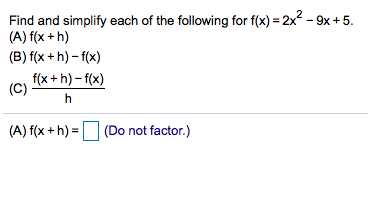# Find and simplify each of the following for f(x) 2x-9x +5(A) f(x +h)(B) f(x +h)-f(x)f(x+h)-f(x)(C)(A) f(x+h)(Do(Do not factor.)

Question
157 viewshelp_outlineImage TranscriptioncloseFind and simplify each of the following for f(x) 2x-9x +5 (A) f(x +h) (B) f(x +h)-f(x) f(x+h)-f(x) (C) (A) f(x+h)(Do (Do not factor.) fullscreen
check_circle

Step 1

The given function is,

Step 2

Compute f (x + h) as follows.

Step 3

Compute f (x + h) – f(x) as fol...

### Want to see the full answer?

See Solution

#### Want to see this answer and more?

Solutions are written by subject experts who are available 24/7. Questions are typically answered within 1 hour.*

See Solution
*Response times may vary by subject and question.
Tagged in
MathCalculus

### Other